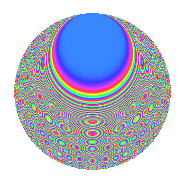# Properties

 Label 2100.2.clLevel 2100 Weight 2 Character orbit cl Rep. character $$\chi_{2100}(557,\cdot)$$ Character field $$\Q(\zeta_{12})$$ Dimension 192 Sturm bound 960

# Related objects

## Defining parameters

 Level: $$N$$ $$=$$ $$2100 = 2^{2} \cdot 3 \cdot 5^{2} \cdot 7$$ Weight: $$k$$ $$=$$ $$2$$ Character orbit: $$[\chi]$$ $$=$$ 2100.cl (of order $$12$$ and degree $$4$$) Character conductor: $$\operatorname{cond}(\chi)$$ $$=$$ $$105$$ Character field: $$\Q(\zeta_{12})$$ Sturm bound: $$960$$

## Dimensions

The following table gives the dimensions of various subspaces of $$M_{2}(2100, [\chi])$$.

Total New Old
Modular forms 2064 192 1872
Cusp forms 1776 192 1584
Eisenstein series 288 0 288

## Trace form

 $$192q - 4q^{7} + O(q^{10})$$ $$192q - 4q^{7} - 24q^{21} - 8q^{31} - 10q^{33} + 8q^{37} - 32q^{43} + 24q^{51} - 60q^{57} + 76q^{61} - 26q^{63} + 16q^{67} + 8q^{73} + 16q^{81} + 38q^{87} + 84q^{91} + 6q^{93} + 40q^{97} + O(q^{100})$$

## Decomposition of $$S_{2}^{\mathrm{new}}(2100, [\chi])$$ into newform subspaces

The newforms in this space have not yet been added to the LMFDB.

## Decomposition of $$S_{2}^{\mathrm{old}}(2100, [\chi])$$ into lower level spaces

$$S_{2}^{\mathrm{old}}(2100, [\chi]) \cong$$ $$S_{2}^{\mathrm{new}}(105, [\chi])$$$$^{\oplus 6}$$$$\oplus$$$$S_{2}^{\mathrm{new}}(210, [\chi])$$$$^{\oplus 4}$$$$\oplus$$$$S_{2}^{\mathrm{new}}(420, [\chi])$$$$^{\oplus 2}$$$$\oplus$$$$S_{2}^{\mathrm{new}}(525, [\chi])$$$$^{\oplus 3}$$$$\oplus$$$$S_{2}^{\mathrm{new}}(1050, [\chi])$$$$^{\oplus 2}$$

## Hecke characteristic polynomials

There are no characteristic polynomials of Hecke operators in the database